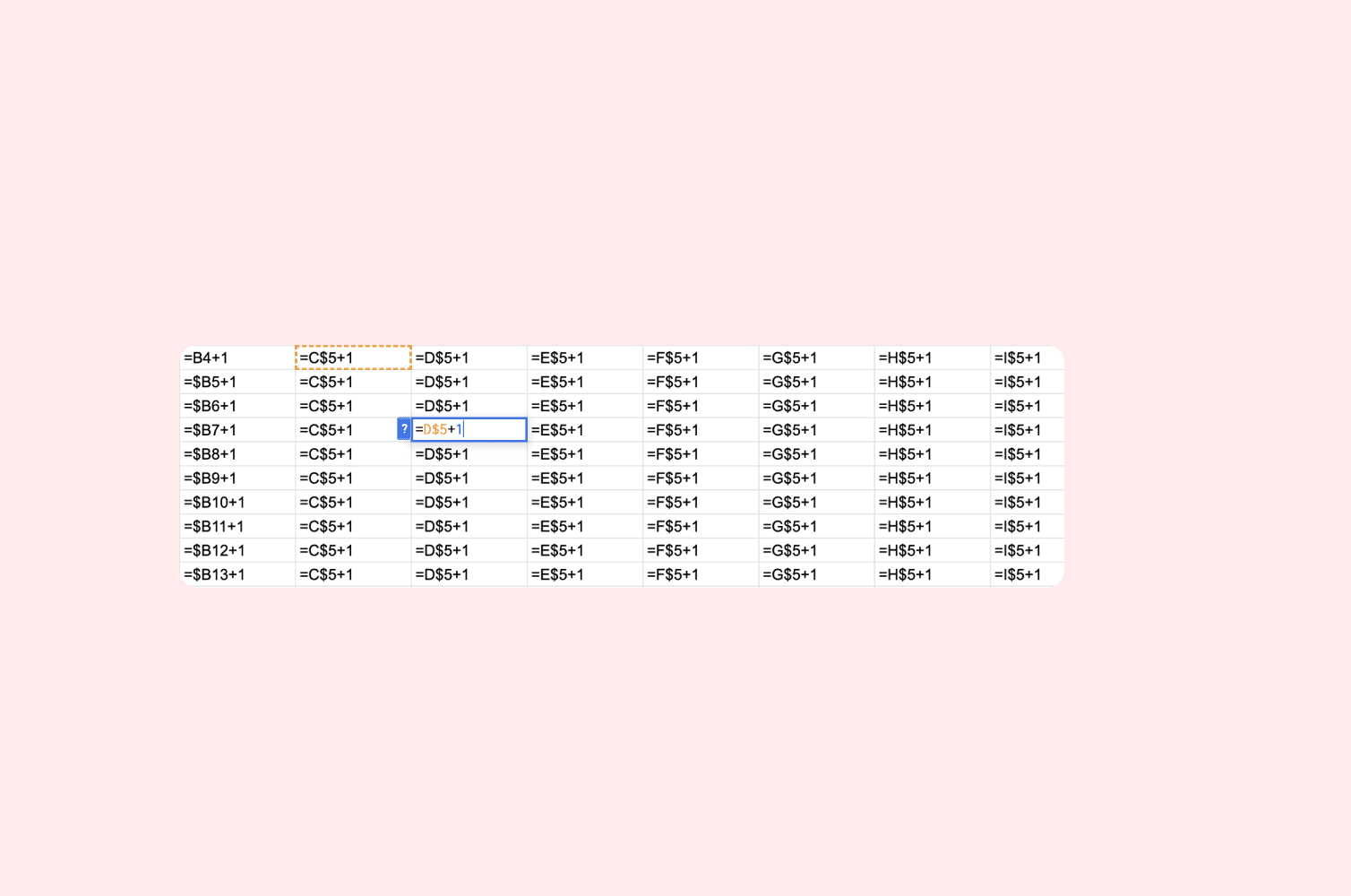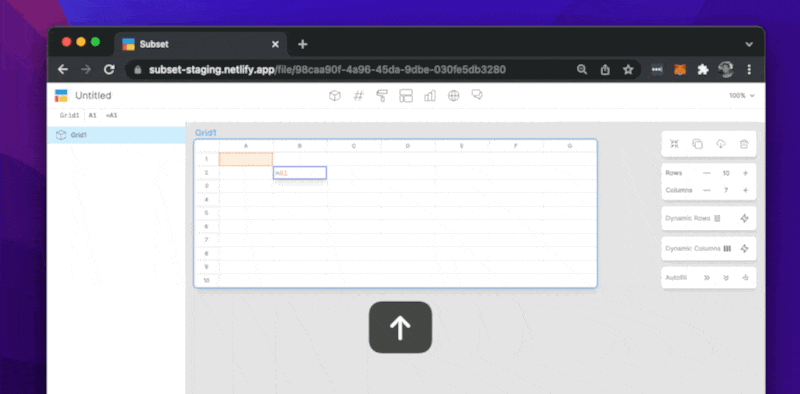How to

# How cell reference locking works

The dollar signs you see in Excel formulas

The dollar signs you see in Excel formulas

The dollar signs you see in Excel formulas

Aug 10, 2022

Aug 10, 2022# How to lock cells in a Spreadsheet?

Have you ever wondered what the difference between `=A1`, `=\$A\$1`, `=A\$1`, and `=\$A1` is in a spreadsheet? While they may look like minor differences, the distinction is what makes spreadsheets one of the most powerful software programs in the world. The \$ represents cell locking in a spreadsheet, and learning about the power of cell locking can 10x your productivity in a spreadsheet.

Before we get into instructions on how to lock cells, it’s important to understand how cells are defined. Spreadsheets typically use the A1 reference format where columns are defined by letters and rows are defined by numbers. Therefore every cell is defined by some combination of a letter(s), then a number. A1 is just the first cell in the most upper right of a spreadsheet, which is on column A and row 1.

Cell locking goes hand in hand with autofill. In a spreadsheet, you can write a formula once and then autofill it right or down (or both right and down). Additionally, if you like a specific formula in a cell, you can copy and paste that formula anywhere else on a spreadsheet.

If that formula that you are autofilling or copying and pasting is referenced to another cell in the file, how do you determine what cells are referenced in all of the new cells you just autofilled or pasted to? That’s where cell locking comes in.

If you don’t lock your cells (meaning there are no \$ signs in your formula), then whenever you autofill or copy and paste, the reference will always maintain the same relationship as your original cell. For example, if you have the formula `=A1` in the cell `A2`, no matter where you autofill or copy and paste to, the reference will always be one cell above, because `A1` is one cell above `A2`.

If you fully lock your cells (meaning there is a \$ before both the column letter and the row number) then the reference will remain unchanged, or locked to the original cell, no matter where you autofill or copy and paste. For example, if you have the formula `=\$A\$1` in the cell `A2`, no matter where you autofill or copy and paste to, the reference will always be =\$A\$1. The current value in `A1` will be what populates in all the other cells with that formula.

Alternatively, you can also just lock one dimension, just the column or row.

Typically there are shortcuts to help you lock references quicker (such as pressing F4 to cycle through the 4 variations). In proper spreadsheet software, there should also be an autofill shortcut to help you copy and paste consecutive rows and columns (often times using CTRL or CMD R for right and D for down).

See the below example in Subset:

`A1` = Nothing is locked

`\$A1` = The column is locked, but the row is unlocked.

`A\$1` = The column is unlocked, but the row is locked.By leveraging the power of cell locking, you can efficiently and accurately autofill formulas throughout your entire spreadsheet.

# How to lock cells in a Spreadsheet?

Have you ever wondered what the difference between `=A1`, `=\$A\$1`, `=A\$1`, and `=\$A1` is in a spreadsheet? While they may look like minor differences, the distinction is what makes spreadsheets one of the most powerful software programs in the world. The \$ represents cell locking in a spreadsheet, and learning about the power of cell locking can 10x your productivity in a spreadsheet.

Before we get into instructions on how to lock cells, it’s important to understand how cells are defined. Spreadsheets typically use the A1 reference format where columns are defined by letters and rows are defined by numbers. Therefore every cell is defined by some combination of a letter(s), then a number. A1 is just the first cell in the most upper right of a spreadsheet, which is on column A and row 1.

Cell locking goes hand in hand with autofill. In a spreadsheet, you can write a formula once and then autofill it right or down (or both right and down). Additionally, if you like a specific formula in a cell, you can copy and paste that formula anywhere else on a spreadsheet.

If that formula that you are autofilling or copying and pasting is referenced to another cell in the file, how do you determine what cells are referenced in all of the new cells you just autofilled or pasted to? That’s where cell locking comes in.

If you don’t lock your cells (meaning there are no \$ signs in your formula), then whenever you autofill or copy and paste, the reference will always maintain the same relationship as your original cell. For example, if you have the formula `=A1` in the cell `A2`, no matter where you autofill or copy and paste to, the reference will always be one cell above, because `A1` is one cell above `A2`.

If you fully lock your cells (meaning there is a \$ before both the column letter and the row number) then the reference will remain unchanged, or locked to the original cell, no matter where you autofill or copy and paste. For example, if you have the formula `=\$A\$1` in the cell `A2`, no matter where you autofill or copy and paste to, the reference will always be =\$A\$1. The current value in `A1` will be what populates in all the other cells with that formula.

Alternatively, you can also just lock one dimension, just the column or row.

Typically there are shortcuts to help you lock references quicker (such as pressing F4 to cycle through the 4 variations). In proper spreadsheet software, there should also be an autofill shortcut to help you copy and paste consecutive rows and columns (often times using CTRL or CMD R for right and D for down).

See the below example in Subset:

`A1` = Nothing is locked

`\$A1` = The column is locked, but the row is unlocked.

`A\$1` = The column is unlocked, but the row is locked.By leveraging the power of cell locking, you can efficiently and accurately autofill formulas throughout your entire spreadsheet.